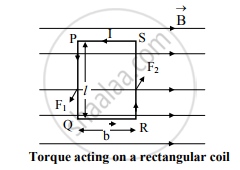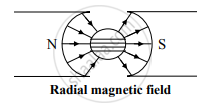Share

# Show that the Current Flowing Through a Moving Coil Galvanometer is Directly Proportional to the Angle of Deflection of Coil. - Physics

#### Question

Show that the current flowing through a moving coil galvanometer is directly proportional to the angle of deflection of coil.

#### Solution

a.   Suppose that rectangular coil PQRS is kept in uniform magnetic field of induction ‘B’. Let ‘n’ be the number of turns of the coil with ‘l’ as its length and ‘b’ as its breadth. The current ‘I’ is passed through it in anticlockwise direction.b.   The forces on QR and SP are equal to zero because they are parallel to B→
c.   Force on PQ,
F1 = nIBl (directed normally outwards)
Force on RS,
F2 = nIBl (directed normally inwards)
d.   Two forces F1 and F2 are equal in magnitude but opposite in direction and act at different points. Hence these forces  constitute a torque (τ) and rotate the coil.
e.   Magnitude of torque is given by,
τ = Magnitude of one of the forces × perpendicular distance between these parallel forces
∴ τ = (nBIl) (b) = nBI (lb)
∴ τ = nBIA

where, A = lb = area of rectangular coil PQRS

f. This torque deflects the coil hence it is called deflecting  torque. It is given by,
τd = nBIA                          ….(i)

This torque causes the pointer attached to the coil to deflect and move on a graduated scale.

g. As the coil is deflected, the phosphor bronze wire is twisted. This twist in phosphor bronze wire provides restoring or controlling torque.h. This restoring torque is directly proportional to the deflection of the coil.
i.e. τr ∝ θ
∴ τr = Cθ                          ….(ii)

where, the constant of proportionality C is called twist constant or restoring torque per unit twist.

i. For equilibrium of the coil, τd = τr

∴ nBIA = Cθ
∴ I = (C/nAB)θ                 .…(iii)

Equation (iii) represents the current flowing through M.C.G

j. For a given M.C.G., n, B, A are constant and for given suspension fibre
C = constant.

∴ C/nAB= k = constant
∴ I = kθ
∴ I ∝ θ
Hence, current flowing through galvanometer is proportional to deflection produced in it.

Is there an error in this question or solution?